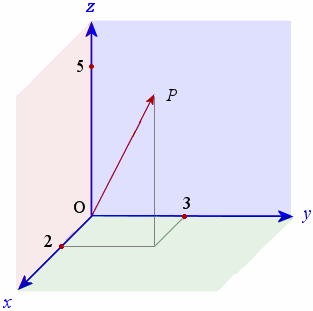## Vectors

A two-dimensional vector, $\bar{v} = \begin{pmatrix}x\\y\end{pmatrix}$, can be thought of representing the displacement that, when starting at the origin, would move something to the point $(x,y)$.

Consequently, a natural interpretation for the sum of two vectors would be the total displacement that results from their individual applications. This can be seen in the picture below, where the red vector represents the total displacement resulting from the application of the blue and green vectors. Fortunately, as seen below, whether we follow the the displacement arising from the blue vector with that from the green vector (the solid segments), or vice-versa (the dashed segments), the overall displacement is the same. (Hence, the addition of vectors is commutative.)As indicated in the picture, this suggests how we might find the sum of two vectors algebraically as well:
$$\begin{pmatrix}x_1\\y_1\end{pmatrix} + \begin{pmatrix}x_2\\y_2\end{pmatrix} = \begin{pmatrix}x_1+x_2\\y_1+y_2\end{pmatrix}$$
Further, understanding how vectors get added together suggests a definition for a constant (or scalar) multiple of a vector. For example, it would be natural to assume that $3\begin{pmatrix}x\\y\end{pmatrix}$ is a displacement three times as large as $\begin{pmatrix}x\\y\end{pmatrix}$. Consequently, $$3\begin{pmatrix}x\\y\end{pmatrix} = \begin{pmatrix}x\\y\end{pmatrix} + \begin{pmatrix}x\\y\end{pmatrix} + \begin{pmatrix}x\\y\end{pmatrix} = \begin{pmatrix}x+x+x\\y+y+y\end{pmatrix} = \begin{pmatrix}3x\\3y\end{pmatrix}$$
In keeping with this example, we define the scalar multiple of a two-dimensional vector, for any real value $c$ (which is called the "scalar" in question), to be
$$c\begin{pmatrix}x\\y\end{pmatrix} = \begin{pmatrix}cx\\cy\end{pmatrix}$$

Of course, vectors need not be two-dimensional. They can have 3, 4, or any positive integer number of dimensions.

As an example, below is an image of the three-dimensional vector $\displaystyle{\begin{pmatrix}2\\3\\5\end{pmatrix}}$The addition and scalar multiplication of these higher-dimensional vectors is defined in a perfectly analogous way. Consider these operations defined three-dimensional vectors: $$\begin{pmatrix}x_1\\y_1\\z_1\end{pmatrix} + \begin{pmatrix}x_2\\y_2\\z_2\end{pmatrix} = \begin{pmatrix}x_1+x_2\\y_1+y_2\\z_1+z_2\end{pmatrix} \quad \textrm{ and } \quad c\begin{pmatrix}x\\y\\z\end{pmatrix} = \begin{pmatrix}cx\\cy\\cz\end{pmatrix}$$